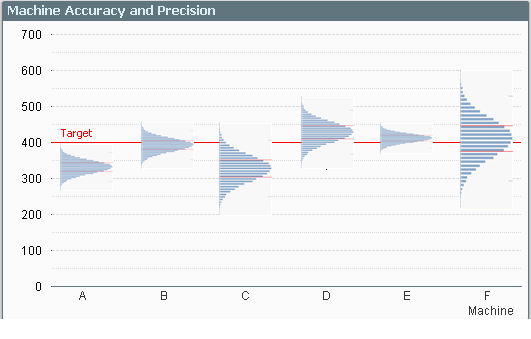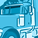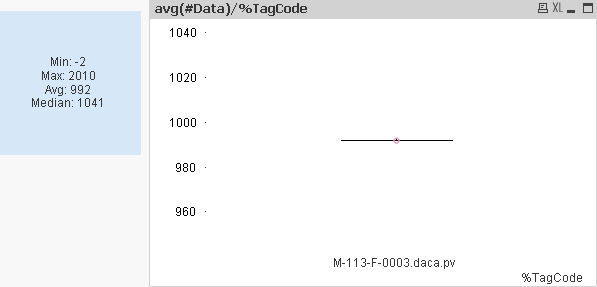# Qlik Design Blog

All about product and Qlik solutions: scripting, data modeling, visual design, extensions, best practices, etc.Employee

## Recipe for a Box Plot

When you want to look at the distribution of a measurement, a histogram is one possibility. However, if you want to show the distribution split over several dimensional values, a Box Plot may be a better choice.

You may, for instance, want to evaluate the quality of units produced in different machines, or delivered by different suppliers. Then, a Box Plot is an excellent choice to display the characteristic that you want to examine:The graph clearly shows you the performance of the different machines compared to target: Machine A has the precision, but not the accuracy. Machine F has the accuracy, but not the precision.

The Box Plot provides an intuitive graphical representation of several properties of the data set. The box itself represents the main group of measurements, with a center line representing the middle of the data. Usually the median and the upper and lower quartile levels are used to define the box, but it is also possible to use the average plus/minus one standard deviation.

The whiskers are used to show the spread of the data, e.g. the largest and smallest measurements can be used. Usually, however, the definition is slightly more intricate. Below I will use the definition used in six sigma implementations.

There, the whiskers are often used to depict the largest and smallest values within an acceptable range, whereas values outside this range are outliers.

The concept of the Inter Quartile Range (IQR) – the difference between the upper and lower quartile level – is used to calculate the acceptance range. Hence:

• Inter Quartile Range (IQR) = Upper Quartile Line (UQL) – Lower Quartile Line (LQL)
• Upper Acceptance Limit (UAL) = UQL + 1.5 * IQR
• Lower Acceptance Limit (LAL) = LQL - 1.5 * IQR

The picture below summarizes the box plot.And here is how you implement this in QlikView…

1. Go to the Tools menu and choose “Box Plot Wizard”.
2. On the “Step 1 - Define data” page, you choose your dimension. In my example, this was Machine, but it could be Supplier or Batch or something similar.
3. Use the same dimension once more in the “Aggregator” control.
4. Use the average of your measurement in the “Expression” control – Avg(Measurement).
5. Click “Next”.
6. On the “Step 2 - Presentation” page, you should choose “Median mode”.
7. Check “Include Whiskers” and “Use Outliers”.
8. Click “Finish”.

QlikView has now created a Box Plot with general expressions that almost always display a meaningful result, and allows for an intermediate aggregator. However, the expressions are not what we want for a six sigma box plot, so we need to change them to the following: (Below, the dimension is called Dim, and the measurement is called Val.)

• Box Plot Middle: Median(Val)
• Box Plot Bottom: Fractile(Val,0.25)
• Box Plot Top: Fractile(Val,0.75)

The whiskers and the outliers all need a nested aggregation – each value needs to be compared to the acceptance levels for the group – so they all contain an Aggr() function that calculates the relevant acceptance limit:

• Box Plot Lower Whisker:
Min(If(Val>= Aggr(2.5*Fractile(total <Dim> Val,0.25) -1.5*Fractile(total <Dim> Val,0.75), Dim, Val), Val))
• Box Plot Upper Whisker:
Max(If(Val<= Aggr(2.5*Fractile(total <Dim> Val,0.75) -1.5*Fractile(total <Dim> Val,0.25), Dim, Val), Val))
• Lower Outlier:
Min(If(Val< Aggr(2.5*Fractile(total <Dim> Val,0.25) -1.5*Fractile(total <Dim> Val,0.75), Dim, Val), Val))
• Upper Outlier:
Max(If(Val> Aggr(2.5*Fractile(total <Dim> Val,0.75) -1.5*Fractile(total <Dim> Val,0.25), Dim, Val), Val))

And with this, I leave you to create your own box plots.

HIC

Further reading related to data classification:

Recipe for a Histogram

Buckets

Recipe for a Pareto AnalysisMVP

Thanks Henric.  My wife is a statistician and SAS programmer, so box plots are everyday things for her.  This is now another thing I can do quicker, easier and better looking in QlikView.6,443 ViewsEmployee

Glad you like it! Just note that my formulae are just one way of doing it: They follow the definitions in Six Sigma. There are other ways to define the five formulae, so you need to find out which definition to use.

HIC

6,443 ViewsSpecialist

Very nice... and useful! Thanks Henric.

6,443 ViewsLuminary Alumni

Given the trend to use small multiples or sparklines, I've always wondered why there isn't a graph that shows mini-distributions instead of the boxplot.  I haven't seen anything like the example below, but I'm curious if anybody has seen anything like it or know why it doesn't exist. .Karl

6,443 ViewsNot applicable

Nice!  Now how about a variable width box plot where the width represents the size of the sample relative to the others.  This is particularly helpful when you show a view across a dimension like your 'machine' example above.

6,443 ViewsPartner

Thanks henric this very nice post. The box polt chart i heard more in sas now i got idea in qlikview.

6,443 ViewsNot applicable

Henrich, is it possible to visualize two boxplots for the same dimension value in the same chart (in a different color)? Adding an extra dimensions seems to distort the boxplot (unless you use trellis but that's not what I want). I cannot add an extra boxplot expression as that option is greyed out.

5,191 ViewsEmployee

No, I cannot get it to work either. I'll check with the developers my this is the case and come back to you.

HIC

5,191 ViewsNot applicable

Amazing! I've been playing around with this and absolutely love it - but I do have one question...

Is it possible to modify this, or the model to select outliers across more than one dimension? For example, lets say you use your cursor to create a box that would select >450 across machines D, E, and F and have say a list of products created on those machines that were outliers be shown in another table. In theory could this be done?

Just curious if someone has thought of this or achieved it, it would be really useful for some of my current work.

5,191 ViewsCreator II

Thanks Henric,

This is a very valuable visualization method.

I applied your recipe (1-8) on measurements and data but it did not work out.I had imported the time series of data via a crosstable

CrossTable(TagCode, #Data)